# CSCI 545 - Python, NumPy, ROS

Fundamentals of the robotics software stack.

Bryon Tjanaka  |  1 September 2021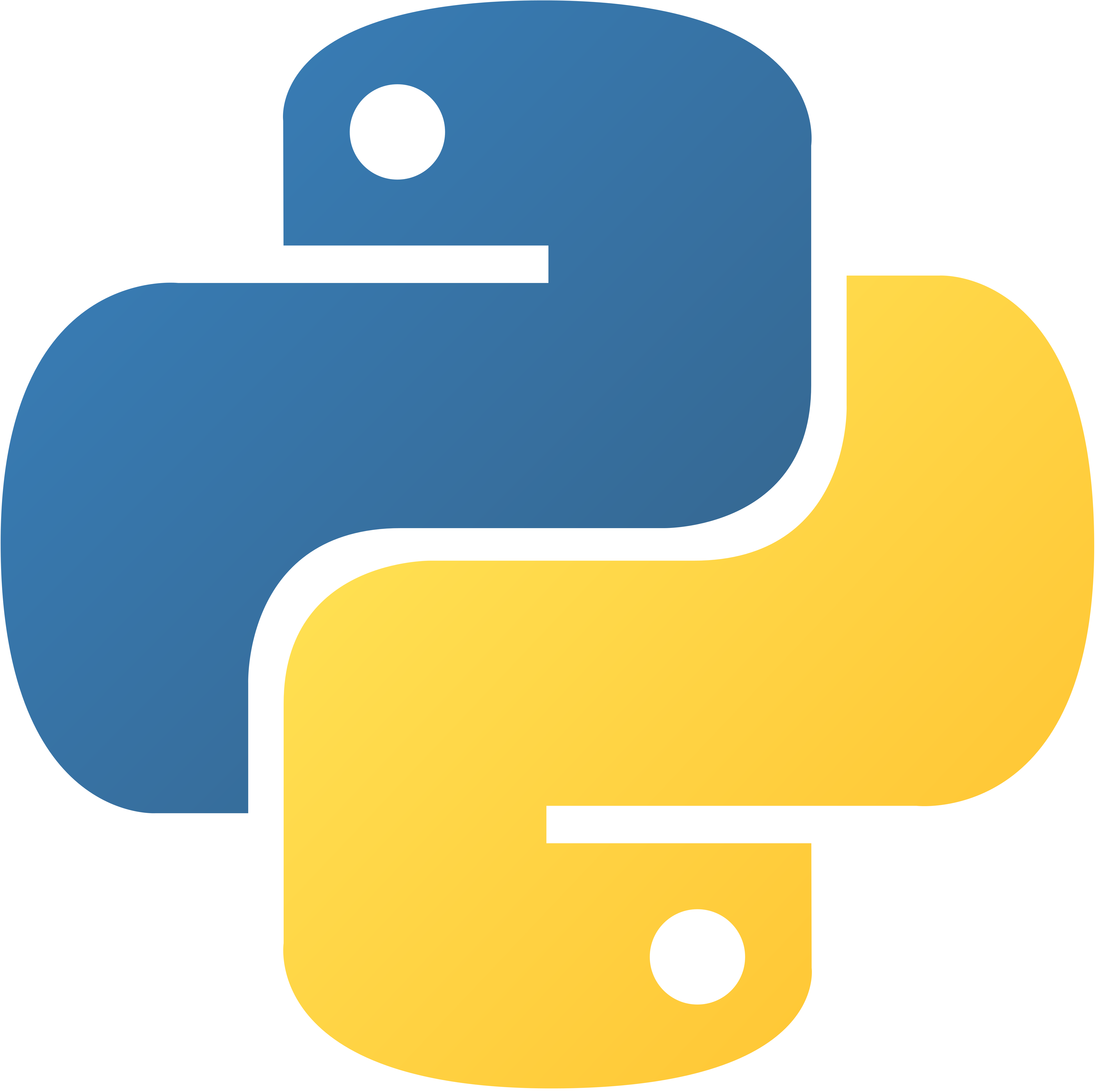# Python at a Glance

• High-level interpreted language
• Great for prototyping
• Slow... but can talk to C/C++/Fortran code
• Mature (30+ years)
• Large ecosystem (e.g. Python scientific stack)
• Popular in robotics research
• Often cited as remarkably similar to pseudocode
• Play around if you are not familiar → Tutorial

# Language Constructs (Demo)

• Assigning variables
• Types: int, float, str, list, tuple, set, dict
• String ops: split, f-strings
• If statements
• For loops: lists, ranges, enumerate
• While loops
• Exception Handling
• Functions and kwargs
• Classes

# Example Programs

``````
def greatest_common_divisor(x, y):
r = x % y
while r > 0:
if r == 0:
print("GCD:", y)
else:
x, y = y, r
``````
``````
import numpy as np  # 12-line neural network.
X = np.array([ [0,0,1],[0,1,1],[1,0,1],[1,1,1] ])
y = np.array([[0,1,1,0]]).T
syn0 = 2*np.random.random((3,4)) - 1
syn1 = 2*np.random.random((4,1)) - 1
for j in xrange(60000):
l1 = 1/(1+np.exp(-(np.dot(X,syn0))))
l2 = 1/(1+np.exp(-(np.dot(l1,syn1))))
l2_delta = (y - l2)*(l2*(1-l2))
l1_delta = l2_delta.dot(syn1.T) * (l1 * (1-l1))
syn1 += l1.T.dot(l2_delta)
syn0 += X.T.dot(l1_delta)
``````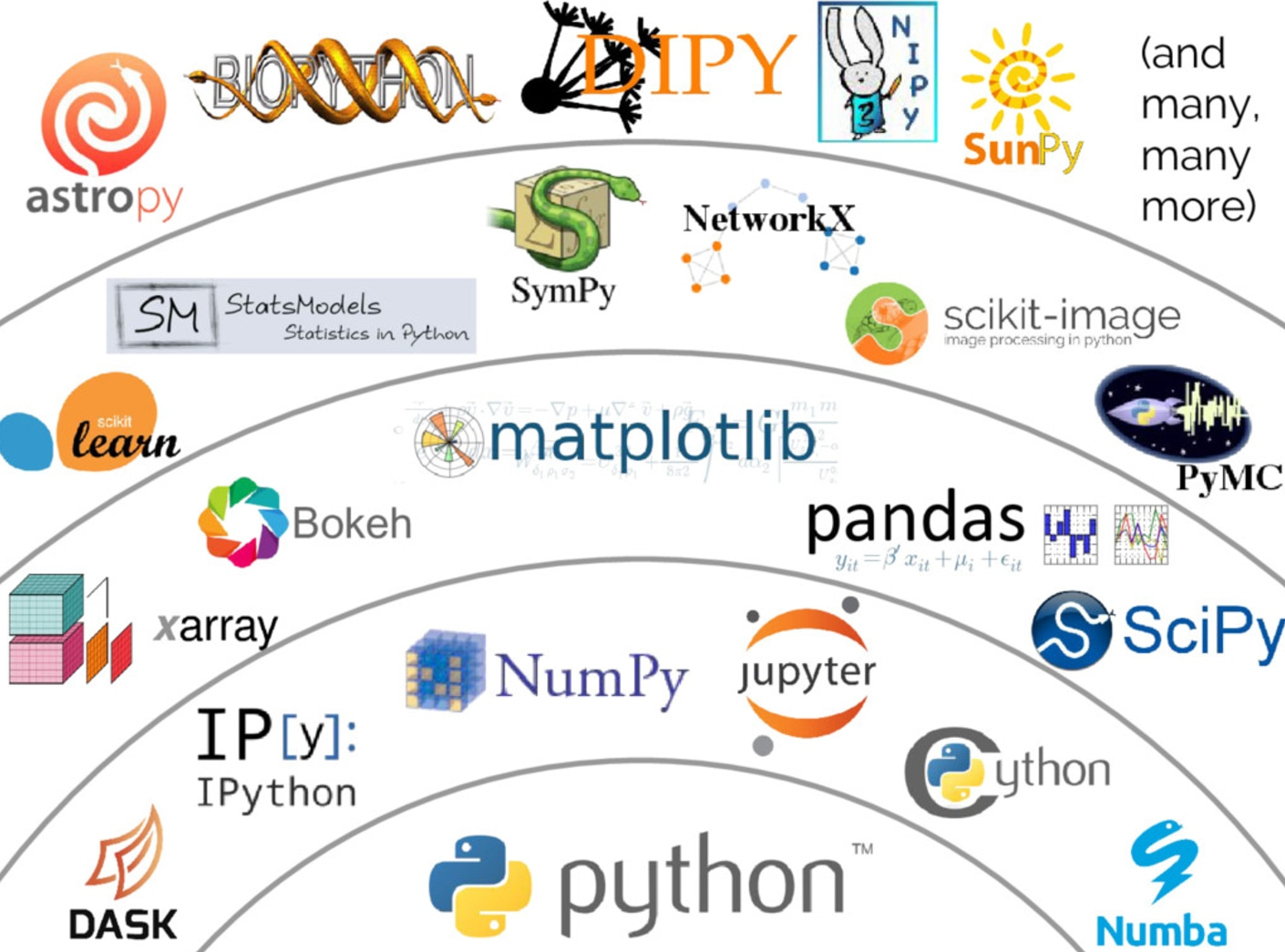# Python Gripes / Problems

• Python = scripting language
• Designed to glue things together
• Problems:
• Slow → JITs like numba can help
• No type checking → Linters like pylint help
• Clunky package management
• Bad concurrency support (search up "Python GIL")

# NumPy provides arrays that are:

• Fast
• Typed
• Fixed-size
• n-dimensional

# Features (Demo)

• Array creation: from lists, ones, zeros, empty, dtype
• Array properties: shape, dtype, ndim
• Indexing
• (Element-wise) arithmetic
• Matrix multiplication
• Randomness: default_rng, standard_normal
• Avoid np.random since it is global

# Robot CommunicationWe need to:

• Connect sensors (e.g. cameras in eyes)
• Command motors (e.g. arms and legs)
• Run various processes (e.g. localization)

# Introducing ROS (Robot Operating System)

• Middleware (not an OS)
• Developed at Willow Garage in 2000s
• Primarily Linux-based
• C++, Python, many other languages
• Facilitates a heterogeneous computer cluster
• Sensors, motors, processors, etc.
• This class: ROS1 with Ubuntu 16.04

# ROS Architecture: Publish/Subscribe

• Nodes: Executables wrapped with ROS magic
• Master node: Name service
• (helps nodes find each other)
• Topics: "Mailboxes" for node data

# Example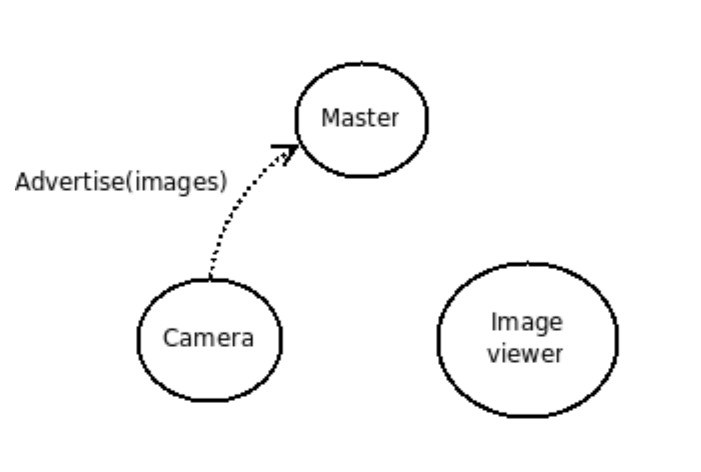Camera wants to publish what it sees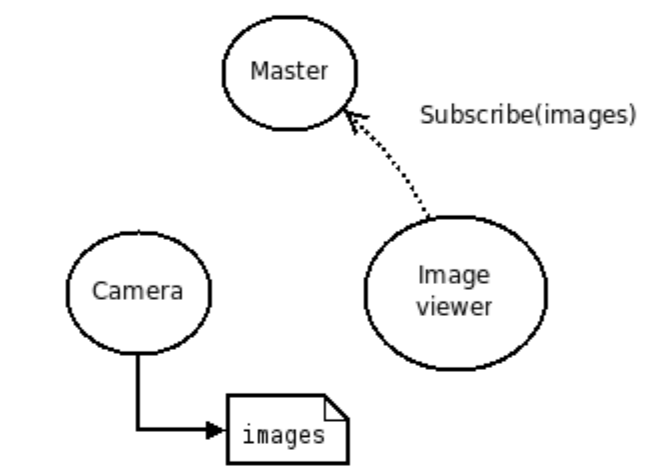Image viewer wants to subscribe to topic "images"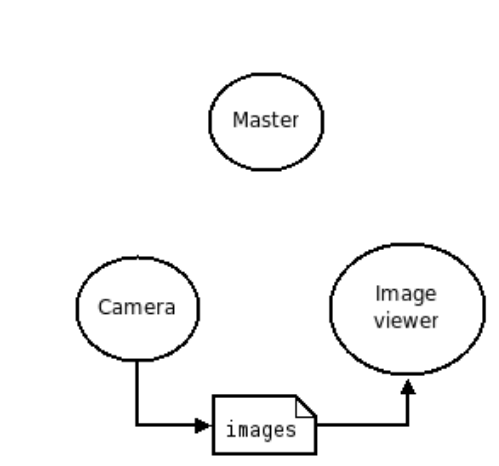Camera sends images to image viewer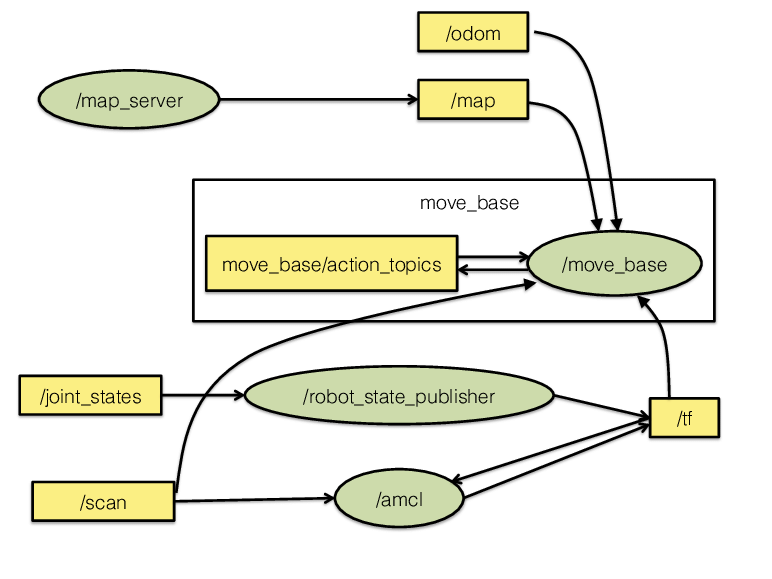# Commands (Demo)

``````
roscore                            # Start master node
rosrun turtlesim turtlesim_node    # Run turtlesim
rosrun turtlesim turtle_teleop_key # Control turtlesim
rosnode list                       # List nodes
rostopic list                      # List topics
rqt_graph                          # View node and topic graph
rosrun rqt_plot rqt_plot           # Plot turtle pose
rviz                               # 3D visualization; we won't
# see anything since this
# is just the turtlesim.
``````

# Miscellaneous

• Catkin: Package and workspace management
• Services: Immediately get a response
• Bagging: Record and replay sessions
• Gazebo: Awesome simulator